# Statistical data

In this section the relevant statistical data for the diffuser computed with Alya is given. The reported data is the one mentioned in Table 2 of the list of desirable quantities (PDF) and also specified here.

The data is available as:

• In CSV (text) format as profiles along characteristic lines of the diffuser
• In CSV (text) format as contours along characteristic planes of the diffuser.
• In HDF5 (binary) format as volumetric data.

### Other turbulent statistics

Other relevant statistics from the database are presented in this section. In particular, the turbulent kinetic energy (TKE) and the characteristic length scales (i.e., the Taylor micro-scale ${\eta _{T}}$and the Kolmogorov length-scale ${\eta _{K}}$) are presented in Fig. 18 along some characteristic contours of the diffuser.

## Profile data

The profile data is thought to match that of the UFR_4-16_Test_Case and the experimental data. Text files (in CSV format) are provided at the streamwise locations x/h=-2, 0, 2, 4, 6, 8, 10, 12, 14, 15.5, 17, 18, 20 and 22 at the following spanwise locations z/B=0.25, 0.5, 0.75 and 0.875. Some of these profiles are also plotted below.

Characteristic Plots of these profiles are: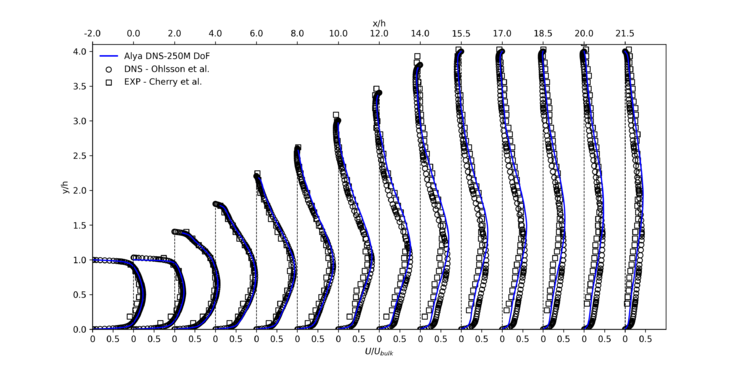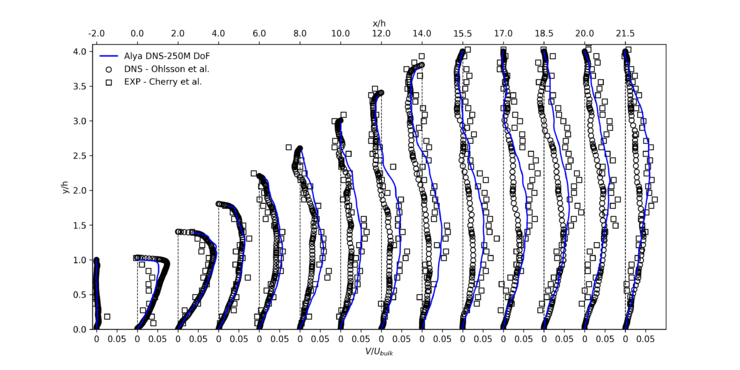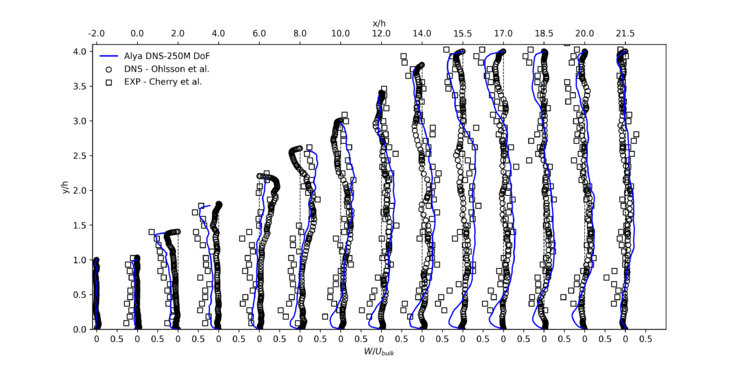Figure 16: Stanford double diffuser, Re=10000, validation. Average velocities at various x/h in the vertical center plane z/B=0.5, Cherry et al. (2008) and Ohlsson et al. (2010) vs data obtained with Alya.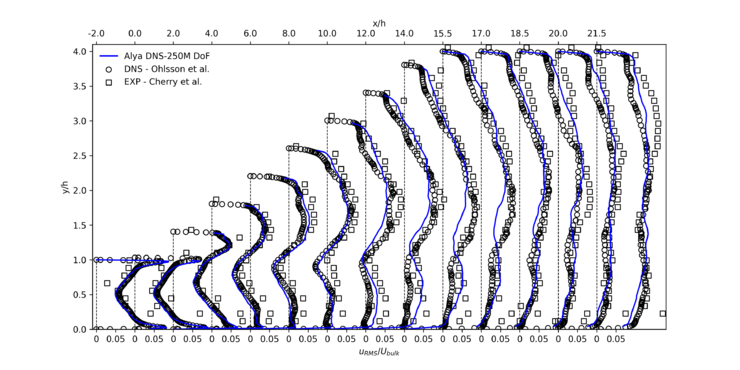Figure 17: Stanford double diffuser, Re=10000, validation. Average streamwise velocity fluctuations at various x/h in the vertical center plane z/B=0.5, Cherry et al. (2008) and Ohlsson et al. (2010) vs data obtained with Alya.

Moreover, budget profiles are provided for the inlet duct area in CSV format for characteristic lines along the y and z directions. These profiles are also plotted below.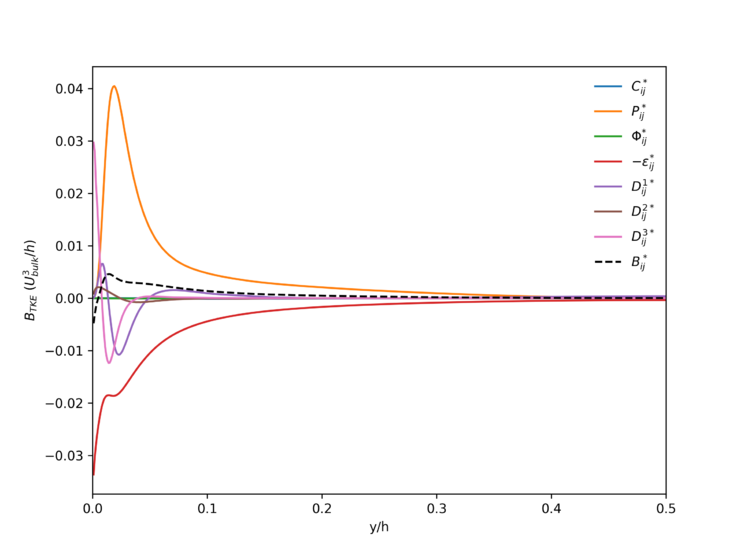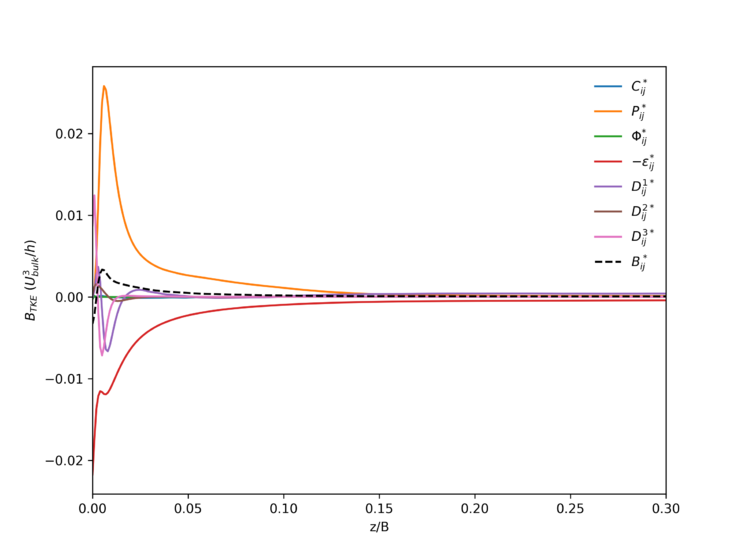Figure 18: Stanford double diffuser, Re=10000. Turbulent kinetic energy budget terms in the inlet duct along the mid-channel lines. The black dashed line is the residual.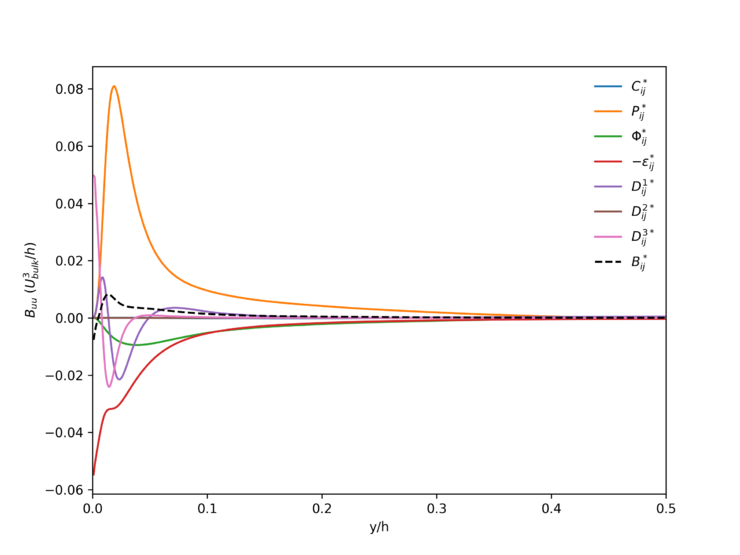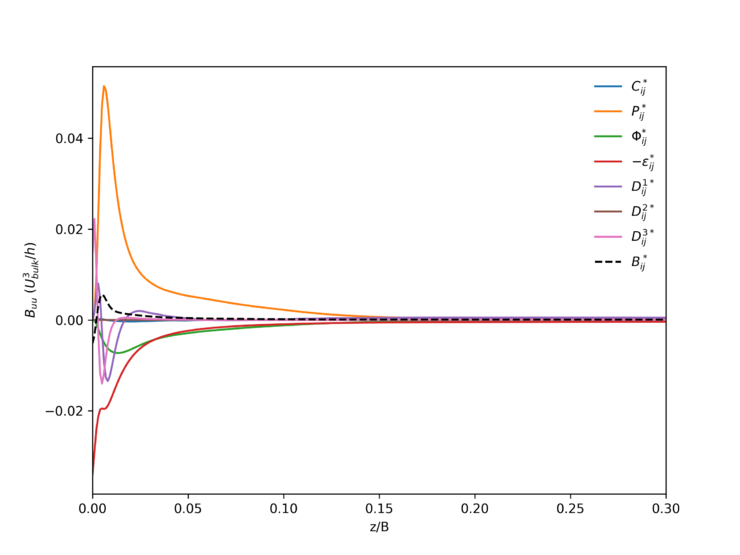Figure 19: Stanford double diffuser, Re=10000. Streamwise Reynolds-stress budget terms in the inlet duct along the mid-channel lines. The black dashed line is the residual.

## Contour data

Contour data is also provided to match that of the UFR_4-16_Test_Case and the experimental data. Text files (in CSV format) are provided at the streamwise locations x/h=2, 5, 8 and 15. These contours are also plotted below.

Characteristic plots of these contours are, on positions x/h=2, 5, 8 and 15: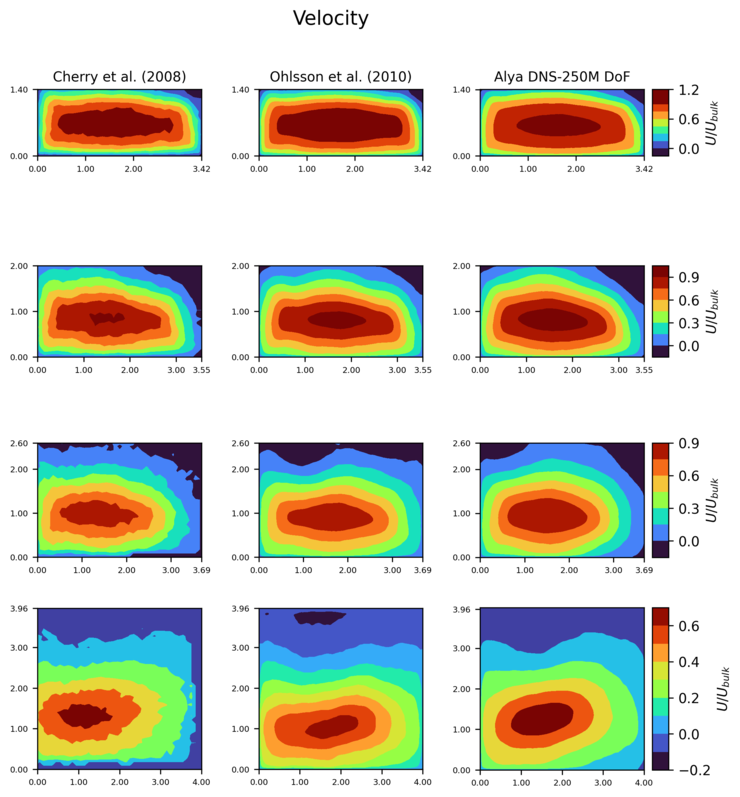Figure 20: Stanford double diffuser, Re=10000, validation. Average streamwise velocity contours, Cherry et al. (2008) and Ohlsson et al. (2010) vs data obtained with Alya. The positions of the contour planes are, from top to bottom, x/h=2, 5, 8 and 15.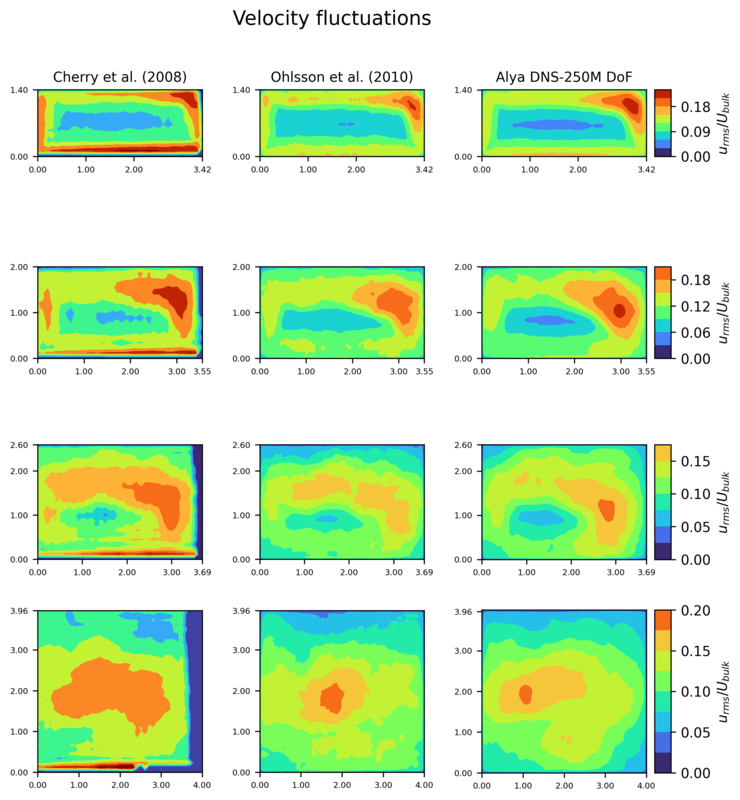Figure 21: Stanford double diffuser, Re=10000, validation. Average streamwise velocity fluctuation contours, Cherry et al. (2008) and Ohlsson et al. (2010) vs data obtained with Alya (smoothed). The positions of the contour planes are, from top to bottom, x/h=2, 5, 8 and 15.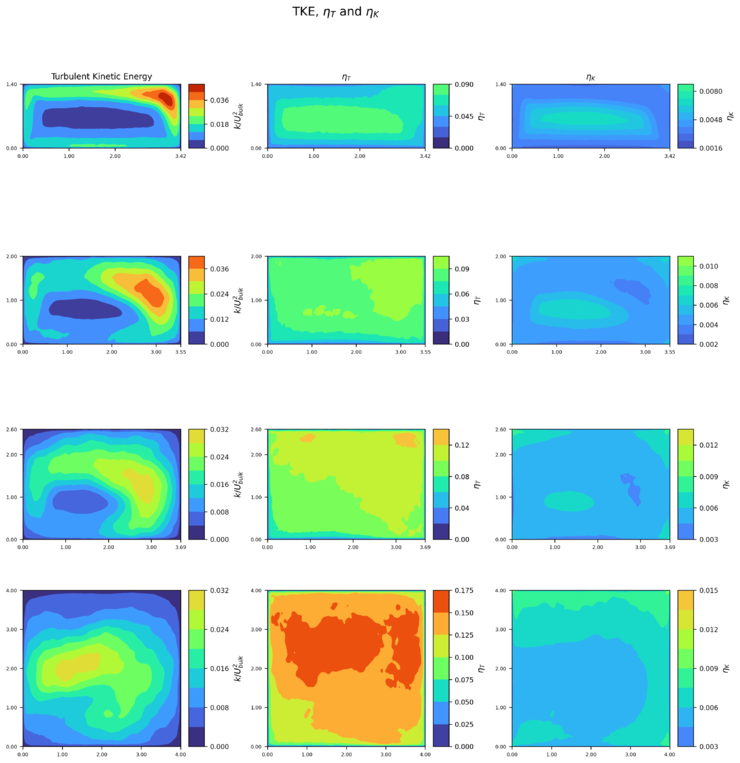Figure 22: Stanford double diffuser, Re=10000. Contours of turbulent kinetic energy (TKE), Taylor microscale and Kolmogorov lengthscale obtained with Alya (smoothed) in planes located, from top to bottom, at x/h=2, 5, 8 and 15.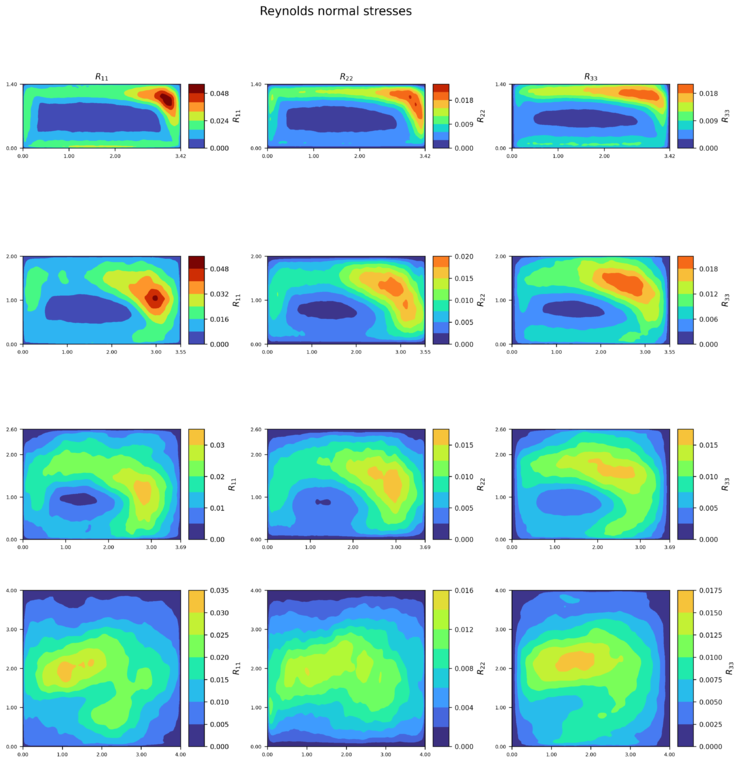Figure 23: Stanford double diffuser, Re=10000. Contours of Reynolds normal stresses (from left to right 11, 22, 33) obtained with Alya (smoothed) in planes located, from top to bottom, at x/h=2, 5, 8 and 15.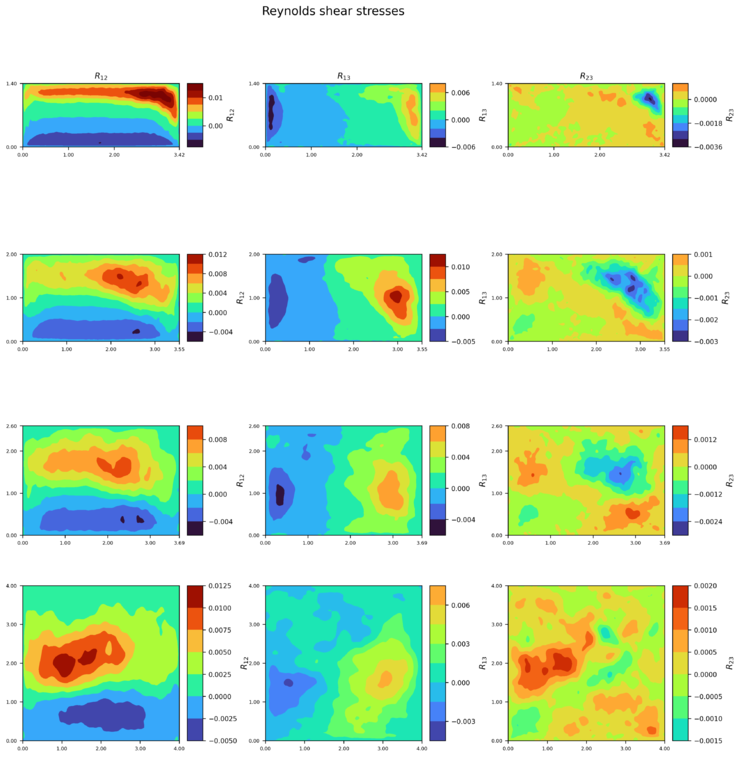Figure 24: Stanford double diffuser, Re=10000. Contours of Reynolds shear stresses (from left to right 12, 13, 23) obtained with Alya (smoothed) in planes located, from top to bottom, at x/h=2, 5, 8 and 15.

## Volume data

Volumetric data on the statistics computed for the whole computational domain are provided here. For more information regarding the storage format, please refer to the storage format guidelines.

The available files are, for the duct area:

And, for the diffuser region:

The following quantities are provided on all four walls as described in this document (PDF):

• Pressure average ${\overline {P}}$• Pressure autocorrelation ${\overline {pp}}$• Shear stress average ${\overline {\tau _{ni}}}$• Shear stress autocorrelation ${\overline {\tau _{ni}'\tau _{nj}'}}$This data is provided in all 4 wall planes of the computational domain

Additionally, the pressure coefficient defined as ${C_{P}=(P-P_{ref})/(0.5\rho U_{bulk})}$, where ${P_{ref}}$is the pressure at ${x/L=0.05}$, is provided along the bottom wall (${y/h=0.0}$) of the diffuser, at ${z/B=0.5}$.

This data has been plotted together with the reference data from Cherry et al. (2008) and Ohlsson et al. (2010). As can be seen, a good agreement is reached with the existing datasets.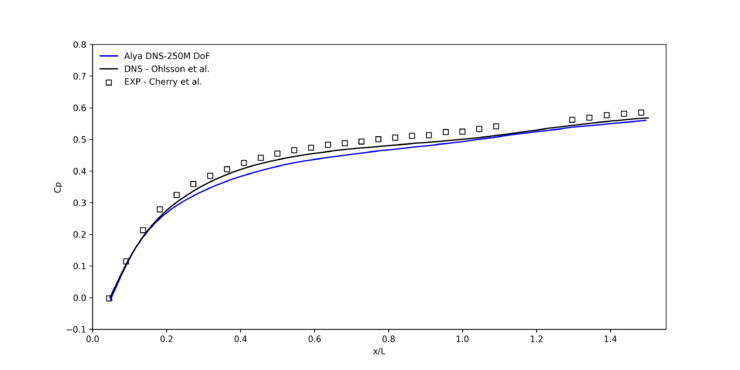Figure 25: Stanford double diffuser, Re=10000, validation. Pressure coefficient along the bottom wall y/h=0 at z/B=0.5, Cherry et al. (2008) and Ohlsson et al. (2010) vs data obtained with Alya.

Contributed by: Oriol Lehmkuhl, Arnau Miro — Barcelona Supercomputing Center (BSC)